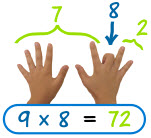# 乘法技巧和窍门

## 最好的窍门

 每个乘数都有个对子，你可能觉得这个对子比较容易记。 例如如果你忘了 8×5，但你可能记得 5×8。这样，你只需记住半个乘法表。## 窍门序列

2

5

6

9是 10× 被乘数，再减被乘数。例如 9×6 = 10×66 = 60−6 = 54

10

11
9x11 或以下： 把数字重复（例如 4x11 = 44）
从 10x11 到 18x11： 把两个位的数字的和放在数字中间

12

## 谨记平方数会有用

 1×1=1 2×2=4 3×3=9 4×4=16 5×5=25 6×6=36 7×7=49 8×8=64 9×9=81 10×10=100 11×11=121 12×12=144

 5×5 = 25 比 6×4 = 24 大 1 6×6 = 36 比 7×5 = 35 大 1 7×7 = 49 比 8×6 = 48 大 1 8×8 = 64 比 9×7 = 63 大 1 等等。。。。。。